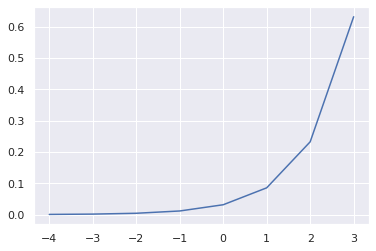Search

# Activation function

Different activation functions are used in the Neural Network (NN) architecture. The work of these activation functions is to activate only the specific neurons responsible for the final prediction. The use of the activation function is to add non-linearity to the NN. The activation can be used in the intermediate layers or at the end of the NN for prediction. The non-linear activation function helps to learn more complex features from the input. The linear activation function can not be used in the intermediate layers of NN as it can not be differentiated. The most commonly used activation functions in the hidden layers are Rectified Linear Activation (ReLU), Logistic (Sigmoid), and Hyperbolic Tangent (Tanh).Activation Function

###### ReLU

ReLU stands for rectified linear activation function. ReLU is better than Sigmoid and Tanh activation function. ReLU helps to overcome the gradient vanishing problem. The range of ReLU function is in max(0.0, x). Only certain neurons are activated at a time. ReLU is very computationally efficient for backpropagation. It suffers from dying ReLU as all the negative outputs are made zero. It accelerates the convergence of the gradients toward global minima due to its linear and non-saturating properties. There are different versions of the ReLU activation function. The leaky ReLU tried to solve the dying ReLU problem by making a small positive slope on the negative left side of the graph. The parametric ReLU function also tried to solve the dying ReLU problem by adding an argument 'a' to the negative left side of the graph. The Exponential linear unit (ELU) activation function also tried to improve the dying ReLU problem by using a log curve on the negative left side of the graph.He Normal and He Uniform:

Xavier Normal and Xavier Uniform (Glorot initialization)

###### Sigmoid

Sigmoid activation is the same as logistic regression function. Its range is in between 0 and 1 using the formula 1.0 / (1.0 + e^-x). The output is not symmetric around zero and all of the output will be of the same sign. This can lead to a vanishing gradient problem. The training of the neural network using Sigmoid is difficult and unstable.###### TanH

It is a hyperbolic tangent activation function. Its range is in between -1 and 1 using the formula f(x) = (e^x – e^-x) / (e^x + e^-x). The tanh function generates very small gradients. It sufferers from vanishing gradient problems as the generated gradient approaches near zero. It helps in centering the data for learning on the next layer. It is preferred to Sigmoid non-linear activation function.ReLU is the most commonly used activation function for multi-perceptron and CNN. Sigmoid and TanH are the most commonly used activation function for Recurrent Neural networks. LSTM uses both Sigmoid and TanH activation functions. A few of the commonly used activation functions used in the output layers are Liner, Sigmoid, and Softmax activation functions.

###### Linear

A linear activation function is also known as an Identity function. It returns the output value the same as the input values using the formula f(x) = x. It does not modify the weighted sum of the input. Normalization and standardization transform is used to scale the target values before passing them to the model.###### Softmax

A softmax activation function is probabilistic values output in the range between 0 and 1 using the formula e^x / sum(e^x). It is a softer version of argmax. For example, the softmax value for a correct predicted class will be near 1 and for the rest of the class will be near 0. The target class will be represented as 0 and 1.The activation function used for the regression is the linear activation function. For the binary classification problem, the Sigmoid activation function is used with one node. For the multi-class classification, the Softmax classification is used with a number of nodes equal to the number of classes. For multi-label classification, the Sigmoid activation function is used with a number of nodes equal to the number of classes.

Creating the NN architecture without using the activation function will create a simple linear regression model. The model will not able to generalize complexity well on the input. It helps to add non-linearly to the model.

## Recent Posts

See All